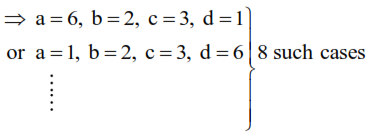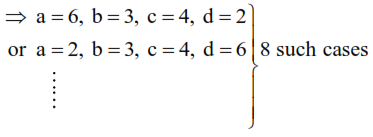# Four dice are thrown simultaneously and the numbers`
Question:

Four dice are thrown simultaneously and the numbers shown on these dice are recorded in $2 \times 2$ matrices. The probability that such formed matrices have all different entries and are non-singular, is :

1. $\frac{45}{162}$

2. $\frac{23}{81}$

3. $\frac{22}{81}$

4. $\frac{43}{162}$

Correct Option: , 4

Solution:

$A=\left|\begin{array}{ll}a & b \\ c & d\end{array}\right| \quad|A|=a d-b c$

Total case $=6^{4}$

For non-singular matrix $|\mathrm{A}| \neq 0 \Rightarrow \mathrm{ad}-\mathrm{bc} \neq 0$

$\Rightarrow \mathrm{ad} \neq \mathrm{bc}$

And $a, b, c, d$ are all different numbers in the set $\{1,2,3,4,5,6\}$

Now for ad $=\mathrm{bc}$

(i) $6 \times 1=2 \times 3$(ii) $6 \times 2=3 \times 4$favourable cases

$={ }^{6} \mathrm{C}_{4}\lfloor 4-16$

required probability

$=\frac{{ }^{6} \mathrm{C}_{4}\lfloor 4-16}{6^{4}}=\frac{43}{162}$